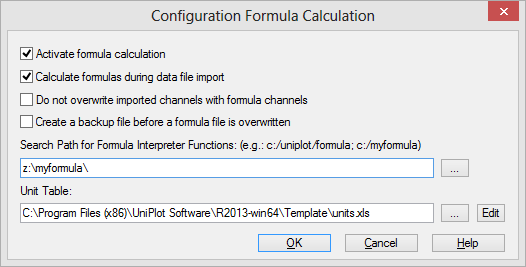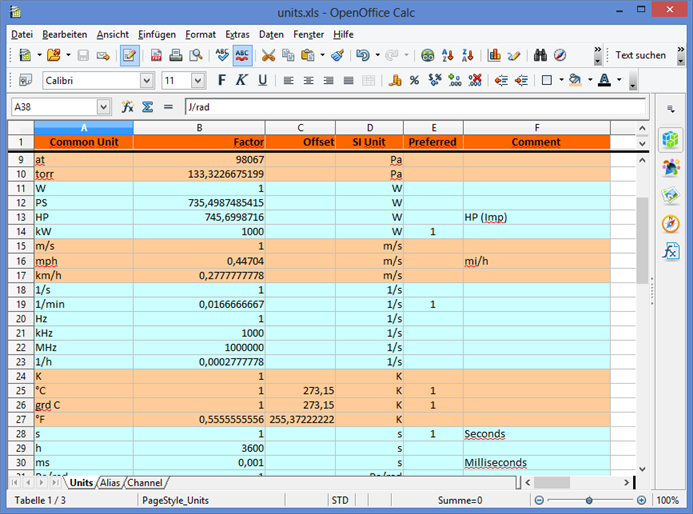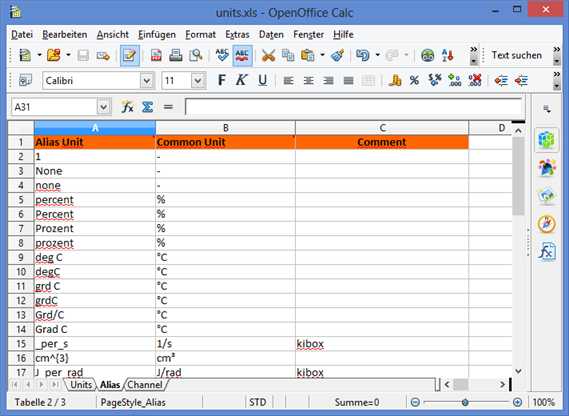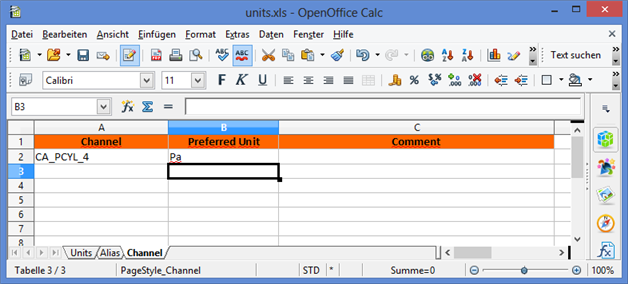# Unit Converter¶

The units can be converted during import (`*.open` to netCDF file `*.nc`) using the linear scaling defined in the unit table. This feature is currently (R2013.11) only available for the KiBox import filter.

The following steps are executed:

• Lookup a given unit in the Alias table. If the unit text does exist in the Alias table the standard unit string is returned. Example: For “J_per_m3rad” the Alias table contains the standard unit “J/(m³ rad)”. If the unit is not listed in the Alias table the search will return the given unit.
• With the result from the Alias table, UniPlot will lookup the preferred unit and scale the data with the specified factor and offset. Example: The alias search returned “kPa”. The preferred unit for “kPa” is “bar”. The data will be converted to “bar”.

The Unit Converter compares strings only to find the scale factor and offset. The search is case sensitive. The unit strings can contain any UNICODE characters. Spaces are not ignored.

If you want to convert `1/s` to `1/min` both units must be specified in the Units table.

## The Units Table¶

The Excel file <uniplot-directory>`\Template\units.xlsx` contains three sheets “Units”, “Alias” and “Channels”. If you would like to modify the file, copy the file into a different folder, for example into the UniPlot user directory (see GetUserDirectory). Otherwise the file will be replaced the next time you install a UniPlot update.

The Excel file name can be set in the Tools=>Formula Configuration dialog box. The file name is saved in the registry in the key `Settings\unit-converter-file`, see also UniPlot’s Use of the Windows Registry.To register the new file location, open the Tools=>Formula Configuration dialog box or open the UniPlot command window and type in the following two commands.

```s = GetUserDirectory() + "Template/units.xlsx"
WriteProfileString("Settings", "unit-converter-file", s);
```

### Units Sheet¶

The table defines the common units and the rule to convert the unit into the SI unit.

The “Units” sheet has 6 columns.It means `Common Unit = Factor * SI-Unit + Offset`, for example `1 mbar = 100 * Pa + 0`.

The “Preferred” column contains the value `1` or must be empty. For each group of units, only one element should be marked with 1. If the column contains the value `1`, the unit will be used as the default unit. For example a channel with pressure data is converted to bar.

### Alias Sheet¶

The table defines alias names for the common units in the units table. The sheet contains three columns:If you want to add an alias to the table, add the alias to the first column and the standard unit to second column “Common Unit”. The name must match one of units in the column “Common Unit” in the “Units” sheet.

### Channel Sheet¶

The table defines the preferred unit for every channel. The sheet contains three columns:Each row contains a channel name, the preferred unit and an optional comment.

A channel name must match the channel in the NC file or may contain placeholders (measuring point identifier). The placeholders are replacements of one up to three numerical values: `<x>` is a placeholder for the values 1 to 9. `<xx>` is a placeholder for the values 01 to 99. `<xxx>` is a placeholder for the values 01 to 999. `x`, `xx` and `xxx` stands for any letter in the range a to z. Example: For the four channels `pcy1`, `pcy2`, `pcy3`, `pcy4` the channel placeholder name is: `pcy<c>`.

The placeholders can be used in the same way to define formulas. See Defining Formulas.

Placeholders should be separated by an underscore or the letter `a` to `z` or `A` to `Z`. Example for `pcy3bank2: pcy<c>bank<b>`.

The following function converts all channels in an NC file to the preferred units (bToSIUnit = `TRUE`) or to SI units (bToSIUnit = `TRUE`): nc_convert_units.

## UniScript Interface¶

The UniScript Interface for the unit conversion is defined in the UniScript file `script\datasets\units.ic`.

Functions Unit Conversion
units units returns the unit converter object.
units_get_other_units units_get_other_units returns for a given unit all available units which have the same SI unit.
units_get_preferred_unit units_get_preferred_unit returns the preferred unit for the given unit or channel name.
units_get_scale units_get_scale returns the factor and offset to convert the data from one unit to the other.
units_get_si_unit units_get_si_unit returns the SI unit for the given unit.

id-2069315